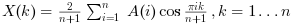Scilab Home page | Wiki | Bug tracker | Forge | Mailing list archives | ATOMS | File exchange
Scilab 6.0.0
Change language to: English - Português - 日本語 - Русский

See the recommended documentation of this function

# dst

Discrete sine transform.

# idst

Inverse discrete sine transform.

### Syntax

```X=dst(A [,sign] [,option])
X=dst(A,sign,selection [,option])
X=dst(A,sign,dims,incr [,option])
X=idst(A [,option])
X=idst(A,selection [,option])
X=idst(A,dims,incr [,option])```

### Arguments

A

a real or complex vector or real or complex array (vector, matrix or N-D array.

X
a real or complex array with same shape as `A`.
sign
an integer. with possible values `1` or `-1`. Select direct or inverse transform. The default value is `-1` (direct transform).
selection
a vector containing index on `A` array dimensions. See the Description part for details.
dims
a vector of positive numbers with integer values, or a vector of positive integers. See the Description part for details.

Each element must be a divisor of the total number of elements of `A`.

The product of the elements must be less than the total number of elements of `A`.

incr
a vector of positive numbers with integer values, or a vector of positive integers. See the Description part for details.

`incr` must have the same number of elements than `dims`.

Each element must be a divisor of the total number of elements of `A`.

The `incr` elements must be in strictly increasing order.

option
a character string. with possible values `"dst1"`, `"dst2"`, `"dst4"` or `"dst"` for direct transform and `"dst1"`, `"dst3"`, `"dst4"` or `"idst"` for inverse transform. The default value is `"dst"` for direct transform and `"idst"` for inverse transform. See the Description part for details.

### Transform description

This function realizes direct or inverse 1-D or N-D Discrete Sine Transforms with shift depending on the `option` parameter value:

• For `"dst1"` the function computes the unnormalized DST-I transform. The 1-D transform of a vector of lengthis:• For `"dst2"` the function computes the unnormalized DST-II transform. the 1-D transform of a vector of lengthis:• For `"dst3"` the function computes the unnormalized DST-III transform. The 1-D transform of a vector of lengthis:• For `"dst4"` the function computes the unnormalized DST-IV transform. the 1-D transform of a vector of lengthis:• For `"dst"` the function computes the normalized DST-I transform. The 1-D transform of a vector of lengthis:• For `"idst"` the function computes the normalized DST-I transform. The 1-D transform of a vector of lengthis:The multi-dimensional DST transforms , in general, are the separable product of the given 1d transform along each dimension of the array. For unnormalized transforms , computing the forward followed by the backward/inverse multi-dimensional transform will result in the original array scaled by the product of the dimension sizes.

### Syntax description

Short syntax
direct

`X=dst(A,-1 [,option])` or `X=dst(A [,option])` gives a direct transform according to the `option` value. The default is normalized DST-I direct transform.

If `A` is a vector (only one dimension greater than 1) a 1-d transform is performed and in the other cases a n-dimensional transform is done.

(the `-1` argument refers to the sign of the exponent..., NOT to "inverse"),

inverse

`X=dst(A,1 [,option])` or `X=idst(A [,option])`performs the inverse transform.

If `A` is a vector (only one dimension greater than 1) a 1-d transform is performed and in the other cases a n-dimensional transform is done.

Long syntax for DST along specified dimensions
• `X=dst(A,sign,selection [,option])` allows to perform efficiently all direct or inverse dst of the "slices" of `A` along selected dimensions.

For example, if `A` is a 3-D array `X=dst(A,-1,2)` is equivalent to:

```for i1=1:size(A,1),
for i3=1:size(A,3),
X(i1,:,i3)=dst(A(i1,:,i3),-1);
end
end```

and `X=dst(A,-1,[1 3])` is equivalent to:

```for i2=1:size(A,2),
X(:,i2,:)=dst(A(:,i2,:),-1);
end```
• `X=dst(A,sign,dims,incr)` is an old syntax that also allows to perform all direct or inverse dst of the slices of `A` along selected dimensions.

For example, if `A` is an array with `n1*n2*n3` elements `X=dst(A,-1,n1,1)` is equivalent to `X=dst(matrix(A,[n1,n2,n3]),-1,1)`. and `X=dst(A,-1,[n1 n3],[1 n1*n2])` is equivalent to `X=dst(matrix(A,[n1,n2,n3]),-1,[1,3])`.

### Optimizing dst

Remark: fftw function automatically stores his last parameters in memory to re-use it in a second time. This improves greatly the time computation when consecutives calls (with same parameters) are performed.

It is possible to go further in dst optimization using get_fftw_wisdom, set_fftw_wisdom functions.

### Algorithms

This function is based on the fftw3 library.

• fft — Transformée de Fourier discrète rapide.
• dct — Discrete cosine transform.
• fftw_flags — choix de la méthode pour la sélection de l'algorithme de planification pour la fft
• get_fftw_wisdom — retourne le wisdom fftw
• set_fftw_wisdom — charge un wisdom fftw
• fftw_forget_wisdom — Réinitialise le wisdom fftw

### Bibliography

Matteo Frigo and Steven G. Johnson, "FFTW Documentation" http://www.fftw.org/#documentation